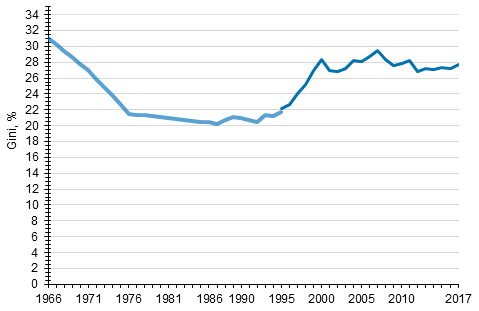Published: 18 December 2018

# Income differentials grew in 2017

Statistics Finland's total statistics on income distribution show that income differentials grew in 2017 compared with the previous year. Real income increased in all income deciles, but more for those with high income than for those with medium and low income. The income level of the population's highest-income decile rose by 4.4 per cent in real terms from 2016 to 2017. The income of medium income earners (income deciles V to IX) increased by 1.3 per cent and the income of the four lowest income deciles by 1.2 per cent. The income level of the lowest-income decile grew by 2.2 per cent.

Change in average real income (%) by income decile from 2016 to 2017Income decile: the population is arranged according to income and divided into ten equal parts, each of which has around half a million persons. The first (I) income decile is the lowest-income decile and the tenth (X) is the highest-income decile. Income concept: equivalent disposable monetary income (incl. realised capital gains) of household dwelling-unit, average per person. Equivalent income: the household-dwelling unit’s income divided by its consumption units (modified OECD-scale).

In 2017, the Gini coefficient describing relative income differentials received the value 27.7, which was 0.5 percentage points higher than in the previous year and almost on level with 2010. Compared with 1995, the Gini coefficient has grown by around 5.5 percentage points. Most of this is caused by the fast growth of income differentials at the end of the 1990s. The income differentials have been at their lowest in the mid-1980s. The Gini coefficient gets the value 0 if everyone receives the same amount of income and 100 if one income earner receives all the income. The higher the Gini coefficient, the bigger the relative income differentials are.

Development of income inequality 1966–2017, Gini coefficient (%)Persons belonging to the household-dwelling population, the household-dwelling unit's equivalent disposable monetary income (incl. realised capital gains). Sources: 1995 to 2017 Total statistics on income distribution; 1986 to 1995: Income distribution statistics (sample); 1966,1971,1976,1981 Household Budget Survey. The missing intervening years (1967 to 1985) have been linearly interpolated.

Source: Total statistics on income distribution, Statistics Finland

Inquiries: Veli-Matti T—rmðlehto 029 551 3680

Director in charge: Jari Tarkoma

Tables

Tables in databases

Updated 18.12.2018

Referencing instructions:

Official Statistics of Finland (OSF): Total statistics on income distribution [e-publication].
Income development by area 2017. Helsinki: Statistics Finland [referred: 11.12.2019].
Access method: http://www.stat.fi/til/tjkt/2017/01/tjkt_2017_01_2018-12-18_tie_001_en.html